# Lecture 16

wjddlstjd396·2022년 2월 16일
0

## CS343-Parallel Programming

목록 보기
13/24

### 6.3.3 Barrier Lock

It is syncronization lock.
It cannot be used for mutual exclusion

The barrier has some state within it.

• some counters
• some flags!

most barriers have internal locking

EX) S1, S2, S3 must all execute before S5, S6, S7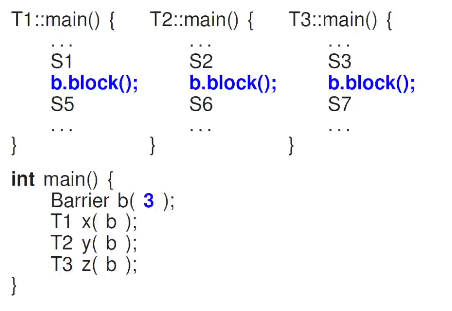in this example, n in b(n) is indicating how many tasks are involved.
so once b.block() is called from 3 threads, barrier will unblock them all.

The lost one to call b.block() must wake other blocked threads.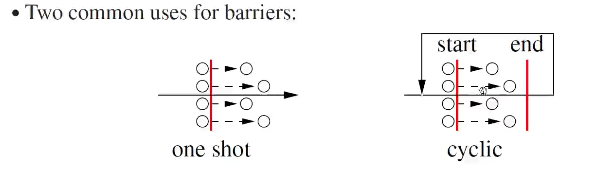many times you want to get the results of the syncronized works.

you can make this work by having coordinator

Barrier start(N+1), end(N+1); //  +1 is for coordinator

//// coordinator's job
start.block(); // wait for threads to start
//do other work
end.block(); // wait for threads to end
// sum up the result
////

//// Workers
start.block()
// do actual work
end.block()
//close down
////

#### 6.3.3.1 uBarrier implimentation

uBarrier is very complicated because it is also a full coroutine

#include <uBarrier.h>
_Cormonitor uBarrier{
protected:
void main(){ for (;;) suspend(); }
virtual void last() {resume();}
public:
uBarrier(unsigned int total);
unsigned int total() const;
unsigned int waiters() const;
void reset(unsigned int total);
virtual void block();
}

unsigned int total is the number of threads involved in the race.
you can change [total] with reset, but only when no threads are on block.

it has internal syncronization lock and no barging.

Need to inherite and refine

• last
• block

members of uBarrier.

EX) matrix sum example - A4 pattern

_Cormonitor Accumulator: public uBarrier{
int total_=0, temp;
protected:
void last(){
temp = total_; total_ = 0;
}
public:
Accumulator(int rows): uBarrier(rows){}
void block(int subtotal){ // this will be working as critical section
total_ += subtotal;
uBarrier::block();
}
int total(){return temp;}
}

int * row, size;
Accumulator & acc;
void main(){
int subtotal = 0;
for(unsigned int r=0; r<size; r++) subtotal += row[r];
acc.block(subtotal); // provide subt total
}
public:
Adder(int row[], int size, Accumulator & acc):
size(size), row(row), acc(acc) {}
};

int main(){
enum {rows = 10, cols = 10};
int matrix[rows][cols];
Accumulator acc(rows); // set berrier with 'rows' number of threads
for(unsigned int r=0; r < rows; r++)
for(unsigned int r=0; r < rows; r++)
cout << acc.total() << " " << acc.Nth() << endl;
}

You cannot use barrier with COFOR
that is because COFOR may create threads by blocks and theres no way it to tell how many of tasks are in a block.

### 6.3.4 Binary Semaphore

Semaphore lock()

lock.P() // to acquire
lock.V() // to release the lock

lock has a counter in it.
initially its 1.
P() will decrement counter
V() will increment counter.

P() on a lock of counter 0 will wait until it is V()ed.

V() on a lock of counter >0 may increment counter above 1 or throw error
this depends on implementation

EX) syncronization with semaphore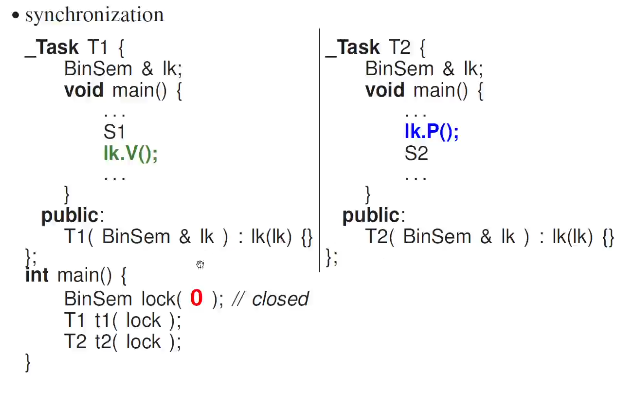EX) mutual exclusion with semaphore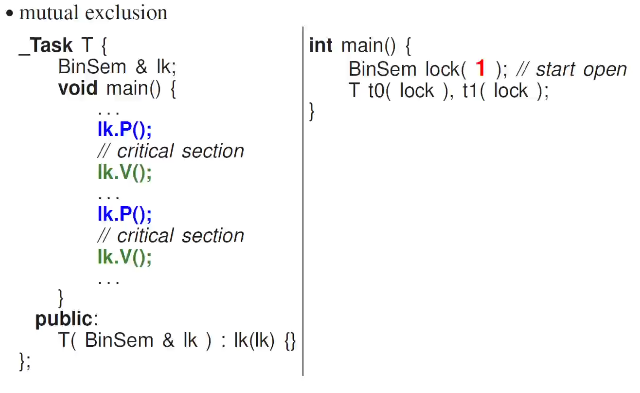Implementation of binary semaphore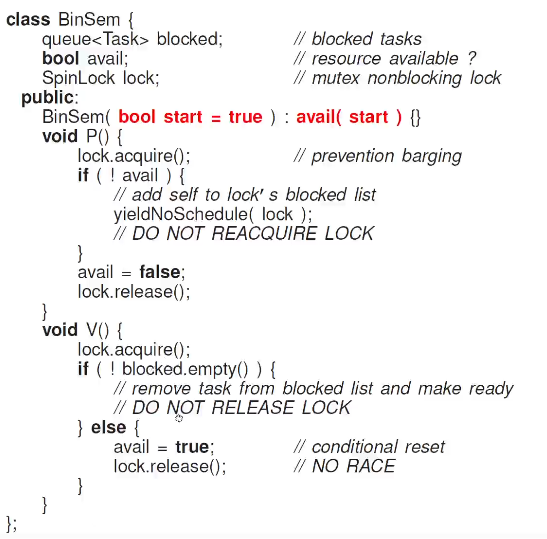Its nearly identical with the lock implementation, but it gives an option to start the lock in either closed or open state

### 6.3.5 Counting Semaphore

A lock with multiple states rather than 0 or 1.

Allows N simultaneous tasks to enter critical sections.